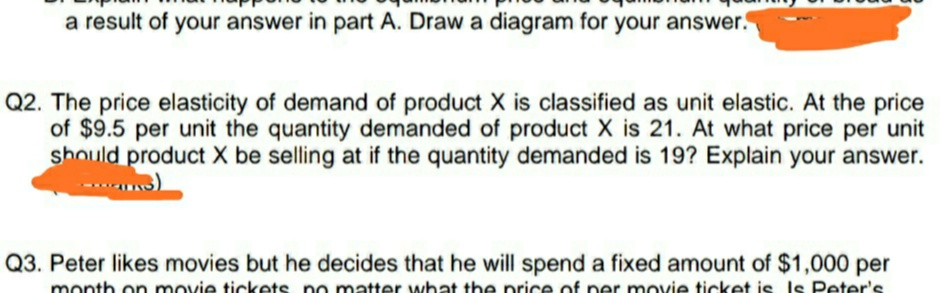# a result of your answer in part A. Draw a diagram for your answer.Q2. The price elasticity of demand of product X is classified as unit elastic. At the priceof \$9.5 per unit the quantity demanded of product X is 21. At what price per unitshould product X be selling at if the quantity demanded is 19? Explain your answer.Q3. Peter likes movies but he decides that he will spend a fixed amount of \$1,000 permonth on movie tickets no matter what the nrice of ner movie ticket is Is Peter's.

Question
10 views

Question is pinned belowhelp_outlineImage Transcriptionclosea result of your answer in part A. Draw a diagram for your answer. Q2. The price elasticity of demand of product X is classified as unit elastic. At the price of \$9.5 per unit the quantity demanded of product X is 21. At what price per unit should product X be selling at if the quantity demanded is 19? Explain your answer. Q3. Peter likes movies but he decides that he will spend a fixed amount of \$1,000 per month on movie tickets no matter what the nrice of ner movie ticket is Is Peter's. fullscreen
check_circle

Step 1

Price elasticity of demand of product X is unit. At price \$9.5 unit per quantity demanded of product X is 21. Since it is unit elasticity, percentage decrease quantity should equal to percentage increase in price.

Step 2

Change in price can be cal...

### Want to see the full answer?

See Solution

#### Want to see this answer and more?

Solutions are written by subject experts who are available 24/7. Questions are typically answered within 1 hour.*

See Solution
*Response times may vary by subject and question.
Tagged in